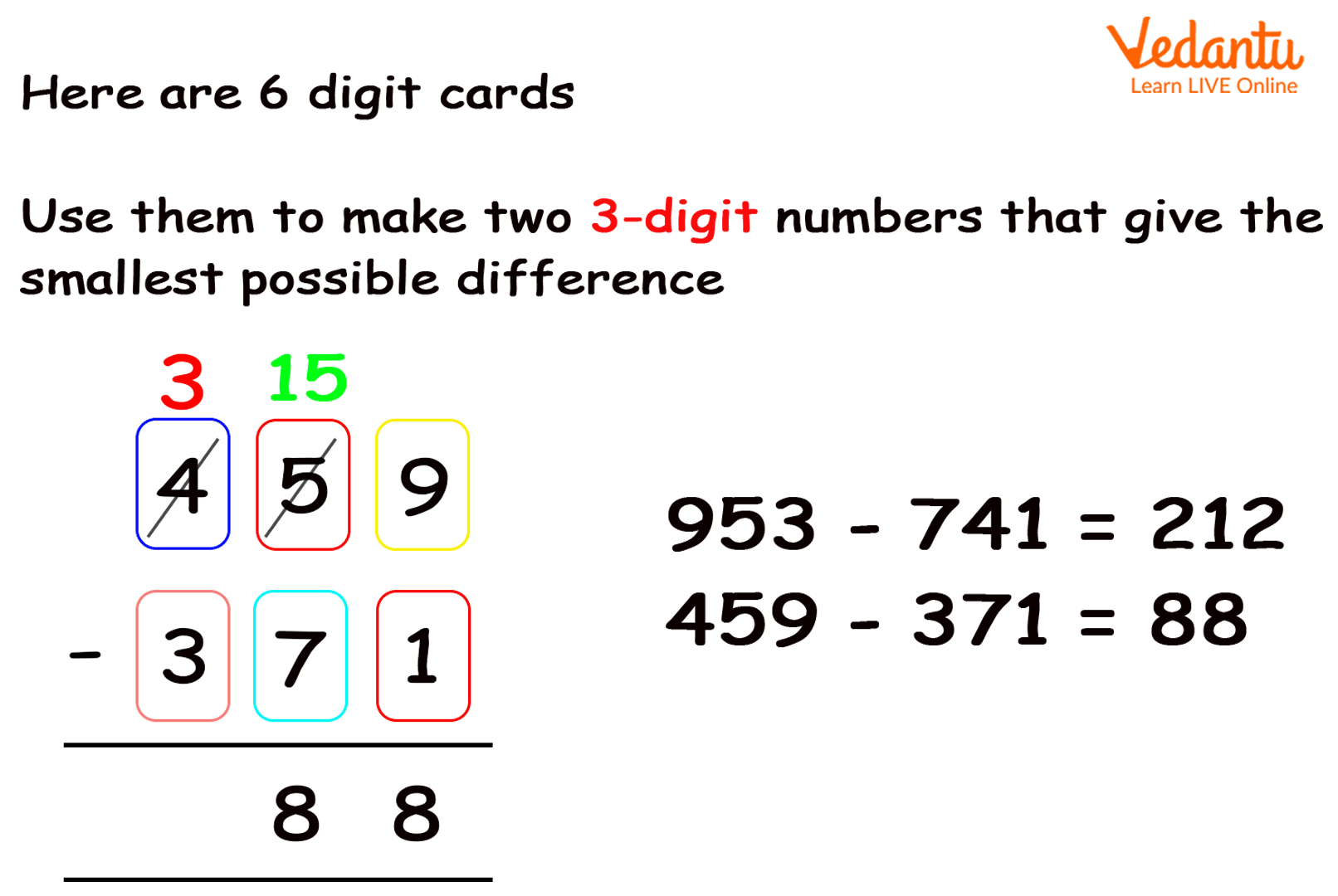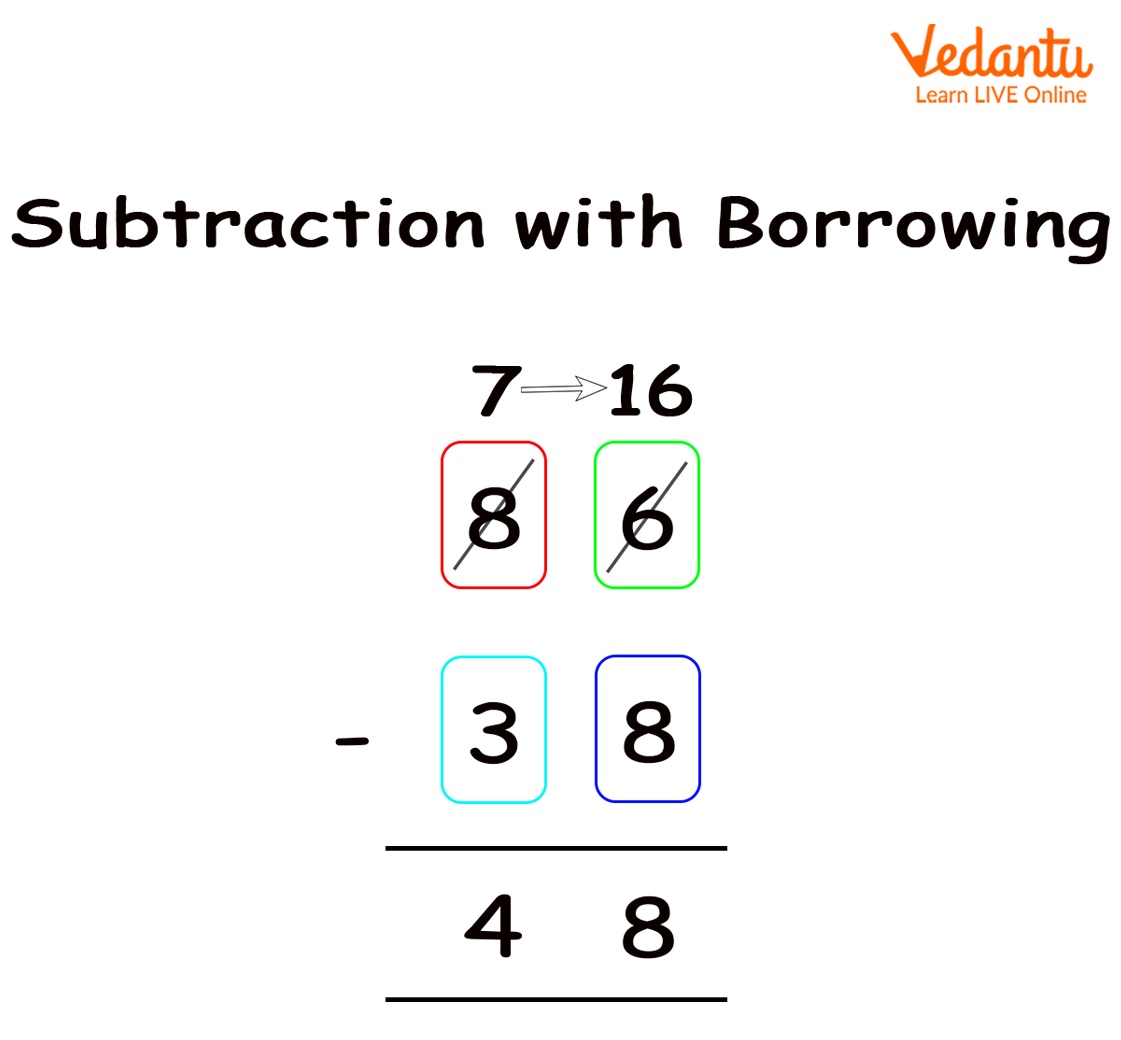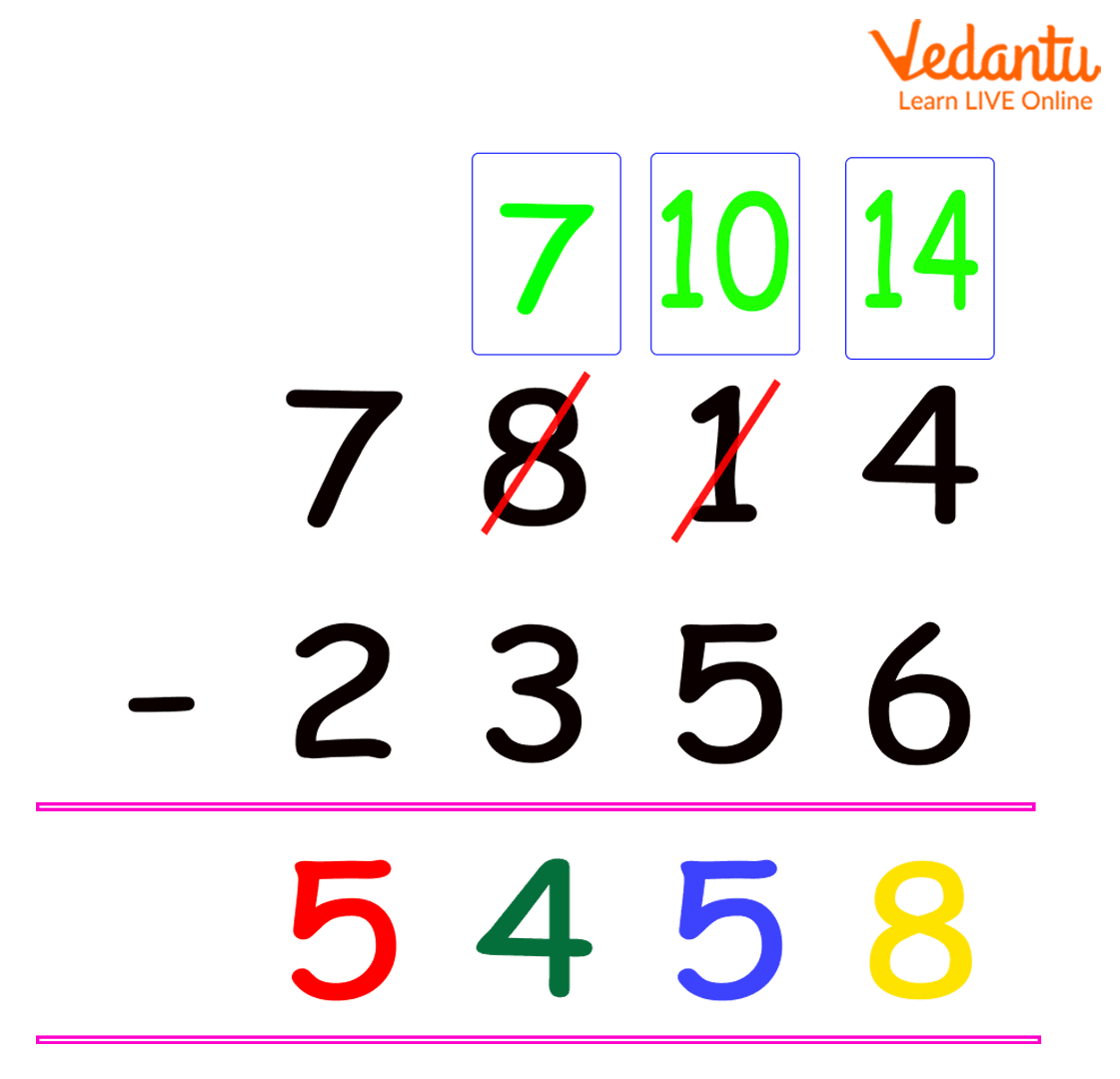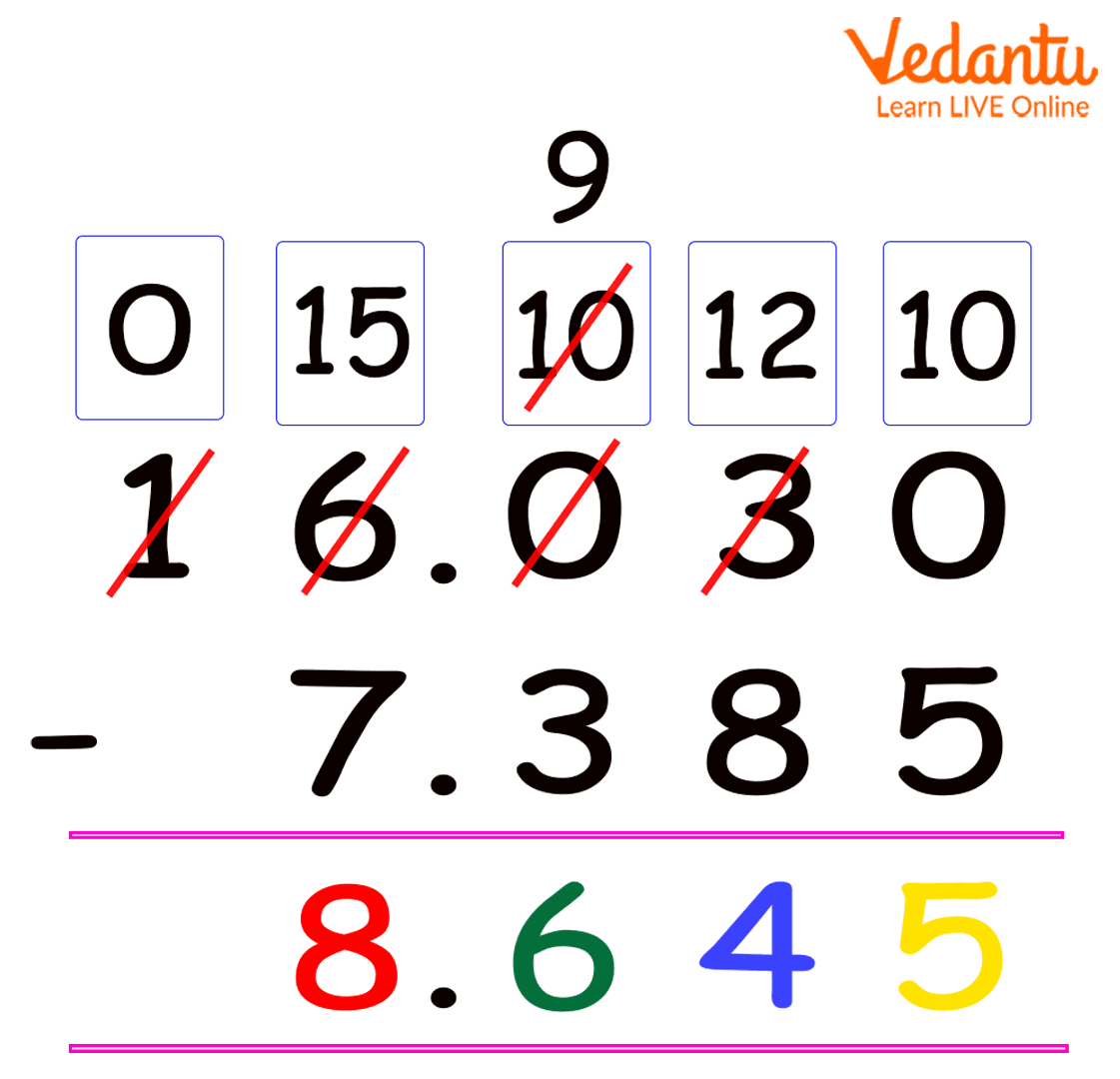Courses
Courses for Kids
Free study material
Free LIVE classes
More

# Difference in MathLIVE
Join Vedantu’s FREE Mastercalss

## Difference in Math - Introduction and Solved Examples

Assume that Neha wanted to buy a new car and she was confused regarding the brand and the model she wanted to purchase. Now, she goes to various showrooms to compare the features, so what does she do? Well, she compares the similarities and differences in features of various models. Similarly, finding the plus and minus is finding the difference.

Why are we talking about this plus-minus or the difference here? Well, the concept of the difference holds in maths as well. So, let us understand the concept of the difference in maths. Here, we will discuss the difference between two-digit, four-digit and decimal numbers with examples.

## Definition of ‘Difference’

The difference is subtracting one number from another, and the process of subtracting or taking away a number from another is called subtraction. Now, the process of subtraction holds the following key terms:

• Subtrahend – The number that is subtracted.

• Minuend – The number from which the subtrahend is subtracted.

• Difference – The result you obtain out of this subtraction is called the difference.

Here, the symbol that we use for subtraction is ‘-’. Also, the formula for finding the difference becomes:

 Minuend – Subtrahend = Difference

For instance, you purchased 20 books from ABC company and gave 13 books to Nina. Now, how many books were left with you?

Well, here the difference between the books is 20 - 13, i.e., 7. Hence, 7 books from ABC company are left with you.

Mathematically, the above problem can be represented as

Number of books you had = 20

Number of books you gave it to Nina = 13

Number of books left with you = 20 - 13 = 7

Here 20 is the minuend, i.e. the number from which another number is being subtracted. 13 is the subtrahend, i.e. the number that is being subtracted. So, the difference or the result is 7.

## Method to Find the Difference between Two Whole or Natural Numbers

Subtraction steps to follow:

The steps to follow in finding the difference between natural numbers or whole numbers are:

1. Place the values vertically as per their place values.

2. Start subtracting the numbers from one’s place.

Let us understand these steps using the following examples:

Example 1: Suppose you want to find the difference between two three-digit numbers, i.e., 459 from 371. Now, let us place one number below the other in order of their place values in the following manner:Difference between two three-digit numbers

Example 2: Suppose, you are asked to find the difference between two four-digit numbers, i.e., 2356 from 7814.

Firstly, we will compare the corresponding digits of both the subtrahend and the minuend.

Secondly, we notice that the value of the subtrahend is larger than the value of the minuend at some places.

Thirdly, a question arises, how do we subtract 4 from 6? So, here, let us understand the concept of borrowing.Subtraction between two two-digit numbers with a borrow

Here, if you see, the one’s digit of 86, i.e., 6 is lesser than the one’s digit of another number 38, i.e., 8, so borrowing 1 from the next number (Here, we borrow 1 from the next number in the place value. ), we get 16 in place of 6 and subtracting 8 from it, we get 8 as the one’s digit in the difference.

Now, going for the ten’s digit, we have 7 in place of 8 and subtracting 3 from 7, we have 4 at the ten’s place in the result.

Thus, taking the one’s and ten’s digit, we get 48 as our answer.

Now, let us get back to our question of 7814 - 2356:Subtraction between two four-digit numbers

Here, we used the same approach as discussed for the difference between 86 and 38.

### Finding the Difference between Decimal Numbers

Example: Subtracting 7.385 from 16.03Subtraction between two decimal numbers

Here also we have used the same approach as discussed for the borrowing concept. Here also, you have to write the numbers in the correct places after decimal.

This was all about finding the difference between whole/natural numbers and decimal numbers.

Last updated date: 01st Oct 2023
Total views: 139.8k
Views today: 4.39k

## FAQs on Difference in Math

1. What is a subtraction example?

In mathematics, subtraction is taking something away from a group of numbers. So, whenever you subtract, a number left in the group becomes less. A simple example of a subtraction problem is the following: 8 - 5 = 3.

2. How do you teach subtraction to your child?

Below are the effective steps to teach a subtraction to your child:

Step 1: Break it up. Start with easy questions of the subtraction.

Step 2: Make him visualise the numbers and strategize.

Step 3: Make him practise the questions till he masters it.

Step 4: Provide a mix of questions to assess his understanding.